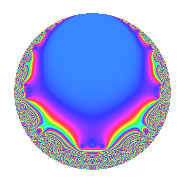# Properties

 Label 27.6.a.bLevel 27 Weight 6 Character orbit 27.a Self dual Yes Analytic conductor 4.330 Analytic rank 1 Dimension 2 CM No Inner twists 1

# Related objects

## Newspace parameters

 Level: $$N$$ = $$27 = 3^{3}$$ Weight: $$k$$ = $$6$$ Character orbit: $$[\chi]$$ = 27.a (trivial)

## Newform invariants

 Self dual: Yes Analytic conductor: $$4.33036313495$$ Analytic rank: $$1$$ Dimension: $$2$$ Coefficient field: $$\Q(\sqrt{17})$$ Coefficient ring: $$\Z[a_1, a_2]$$ Coefficient ring index: $$3$$ Fricke sign: $$1$$ Sato-Tate group: $\mathrm{SU}(2)$

## $q$-expansion

Coefficients of the $$q$$-expansion are expressed in terms of $$\beta = \frac{1}{2}(1 + 3\sqrt{17})$$. We also show the integral $$q$$-expansion of the trace form.

 $$f(q)$$ $$=$$ $$q + ( -4 - \beta ) q^{2} + ( 22 + 9 \beta ) q^{4} + ( -41 + 10 \beta ) q^{5} + ( 5 - 18 \beta ) q^{7} + ( -302 - 35 \beta ) q^{8} +O(q^{10})$$ $$q + ( -4 - \beta ) q^{2} + ( 22 + 9 \beta ) q^{4} + ( -41 + 10 \beta ) q^{5} + ( 5 - 18 \beta ) q^{7} + ( -302 - 35 \beta ) q^{8} + ( -216 - 9 \beta ) q^{10} + ( -277 + 32 \beta ) q^{11} + ( -316 - 72 \beta ) q^{13} + ( 664 + 85 \beta ) q^{14} + ( 1834 + 189 \beta ) q^{16} + ( -24 - 168 \beta ) q^{17} + ( -1474 + 108 \beta ) q^{19} + ( 2518 - 59 \beta ) q^{20} + ( -108 + 117 \beta ) q^{22} + ( -2 + 40 \beta ) q^{23} + ( 2356 - 720 \beta ) q^{25} + ( 4000 + 676 \beta ) q^{26} + ( -6046 - 513 \beta ) q^{28} + ( -6034 - 172 \beta ) q^{29} + ( -793 + 522 \beta ) q^{31} + ( -4854 - 1659 \beta ) q^{32} + ( 6480 + 864 \beta ) q^{34} + ( -7045 + 608 \beta ) q^{35} + ( 3962 + 1080 \beta ) q^{37} + ( 1792 + 934 \beta ) q^{38} + ( -918 - 1935 \beta ) q^{40} + ( -3878 + 2068 \beta ) q^{41} + ( -382 + 1548 \beta ) q^{43} + ( 4850 - 1501 \beta ) q^{44} + ( -1512 - 198 \beta ) q^{46} + ( 1034 - 3184 \beta ) q^{47} + ( -4470 + 144 \beta ) q^{49} + ( 17936 + 1244 \beta ) q^{50} + ( -31576 - 5076 \beta ) q^{52} + ( -1179 - 2178 \beta ) q^{53} + ( 23517 - 3762 \beta ) q^{55} + ( 22430 + 5891 \beta ) q^{56} + ( 30672 + 6894 \beta ) q^{58} + ( 33124 + 1072 \beta ) q^{59} + ( -26104 + 2304 \beta ) q^{61} + ( -16664 - 1817 \beta ) q^{62} + ( 23770 + 7101 \beta ) q^{64} + ( -14404 - 928 \beta ) q^{65} + ( -18406 - 5364 \beta ) q^{67} + ( -57984 - 5424 \beta ) q^{68} + ( 5076 + 4005 \beta ) q^{70} + ( 19560 + 4728 \beta ) q^{71} + ( 24905 - 2592 \beta ) q^{73} + ( -56888 - 9362 \beta ) q^{74} + ( 4508 - 9918 \beta ) q^{76} + ( -23273 + 4570 \beta ) q^{77} + ( -24664 - 288 \beta ) q^{79} + ( -3374 + 12481 \beta ) q^{80} + ( -63072 - 6462 \beta ) q^{82} + ( 47867 + 6560 \beta ) q^{83} + ( -62856 + 4968 \beta ) q^{85} + ( -57296 - 7358 \beta ) q^{86} + ( 41094 - 1089 \beta ) q^{88} + ( 23046 - 10236 \beta ) q^{89} + ( 47668 + 6624 \beta ) q^{91} + ( 13636 + 1222 \beta ) q^{92} + ( 116856 + 14886 \beta ) q^{94} + ( 101474 - 18088 \beta ) q^{95} + ( 91823 - 13680 \beta ) q^{97} + ( 12408 + 3750 \beta ) q^{98} +O(q^{100})$$ $$\operatorname{Tr}(f)(q)$$ $$=$$ $$2q - 9q^{2} + 53q^{4} - 72q^{5} - 8q^{7} - 639q^{8} + O(q^{10})$$ $$2q - 9q^{2} + 53q^{4} - 72q^{5} - 8q^{7} - 639q^{8} - 441q^{10} - 522q^{11} - 704q^{13} + 1413q^{14} + 3857q^{16} - 216q^{17} - 2840q^{19} + 4977q^{20} - 99q^{22} + 36q^{23} + 3992q^{25} + 8676q^{26} - 12605q^{28} - 12240q^{29} - 1064q^{31} - 11367q^{32} + 13824q^{34} - 13482q^{35} + 9004q^{37} + 4518q^{38} - 3771q^{40} - 5688q^{41} + 784q^{43} + 8199q^{44} - 3222q^{46} - 1116q^{47} - 8796q^{49} + 37116q^{50} - 68228q^{52} - 4536q^{53} + 43272q^{55} + 50751q^{56} + 68238q^{58} + 67320q^{59} - 49904q^{61} - 35145q^{62} + 54641q^{64} - 29736q^{65} - 42176q^{67} - 121392q^{68} + 14157q^{70} + 43848q^{71} + 47218q^{73} - 123138q^{74} - 902q^{76} - 41976q^{77} - 49616q^{79} + 5733q^{80} - 132606q^{82} + 102294q^{83} - 120744q^{85} - 121950q^{86} + 81099q^{88} + 35856q^{89} + 101960q^{91} + 28494q^{92} + 248598q^{94} + 184860q^{95} + 169966q^{97} + 28566q^{98} + O(q^{100})$$

## Embeddings

For each embedding $$\iota_m$$ of the coefficient field, the values $$\iota_m(a_n)$$ are shown below.

For more information on an embedded modular form you can click on its label.

Label $$\iota_m(\nu)$$ $$a_{2}$$ $$a_{3}$$ $$a_{4}$$ $$a_{5}$$ $$a_{6}$$ $$a_{7}$$ $$a_{8}$$ $$a_{9}$$ $$a_{10}$$
1.1
 2.56155 −1.56155
−10.6847 0 82.1619 25.8466 0 −115.324 −535.963 0 −276.162
1.2 1.68466 0 −29.1619 −97.8466 0 107.324 −103.037 0 −164.838
 $$n$$: e.g. 2-40 or 990-1000 Significant digits: Format: Complex embeddings Normalized embeddings Satake parameters Satake angles

## Inner twists

This newform does not admit any (nontrivial) inner twists.

## Atkin-Lehner signs

$$p$$ Sign
$$3$$ $$1$$

## Hecke kernels

This newform can be constructed as the kernel of the linear operator $$T_{2}^{2} + 9 T_{2} - 18$$ acting on $$S_{6}^{\mathrm{new}}(\Gamma_0(27))$$.